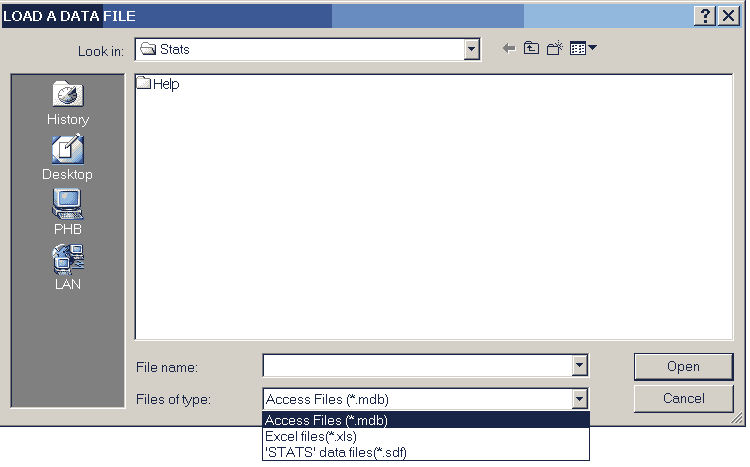STATS commands, page 1The items on this page are:-

CLEARING DATA

clear_all
Clears all data to zero.

b_clear(first column number, last column number)
Clears the block of columns to zero.
e.g. b_clear(3,6) will zero all values in columns 3 through to 6.

DATA STORAGE

save_data( first column number,second column number)
This saves the block of data defined by the two column numbers.
e.g. save_data(1,4) will save all the data in columns 1 to 4.

load_data(column number)
This loads a block of data, starting the storage at the specified column number. The data is added below any existing data in the columns concerned,
e.g. load_data(2) will load a block of data, starting the storage in column 2 and continuing for the number of columns in the block.

If STATS data is being re-loaded, a parameter of zero will re-load it into the same columns from which it was saved. Otherwise, it's loaded into the specified column onwards. A parameter of zero will give an error with Access or Excel data.

Executing the command first displays the data file selection screen.Three types of data file can be loaded.

• MS Access databases, (.MDB files),
• MS Excel workbooks, (.XLS files), and
• STATS custom datafiles, (.SDF files).

DATA PRODUCTION

enter_data(number of items, first column number, last column number)
Allows the specified number of items to be entered from the keyboard into a block of columns, a row at a time.
e.g. enter_data(50,1,3) will allow the entry of 50 rows of data into columns 1 to 3.

If data already exists in the block the entered data is added to the existing so, if this is not wanted, clear_all or b_clear should first be used to remove the existing data.
Clicking the CANCEL button will stop the process; saving the last complete row of data.

seq(initial value,geometric factor,arithmetic factor,number of items,column number)
This will produce a sequence of numbers in the specified column using the first three parameters in a recurence relationship of the form un+1 = g un + a where u1 is the initial value and g and a the geometric and arithmetic factors.
e.g. seq(1,2,1,7,4) will produce the sequence 1, 3, 7, 15, 31, . . . in column 4.

fib(initial value,second value,first term factor,second term factor,number of items,column number)
This will produce a sequence of numbers in the specified column using a recurence relationship of the form un+2 = a un + b un+1 where u1 is the initial value, u2 is the second value, a is the first term factor and b is the second term factor.
e.g. fib(1,2,1,1,20,1) will produce the Fibonacci sequence, 1, 2, 3, 5, 8, 13 . . ., for 20 terms in column 1.

function(function,start value,end value,step value ,x-data column number,function column number)
This will produce a sequence of numbers where the values in the column correspond to the X-data generated by the start value, the end value and the step value and the Function column contains the corresponding values of the function.
The function must be entered with 'x' as the variable using standard BASIC syntax.
e.g. function(x^2,1,8,2,1,3) will produce the values 1,3,5,7 in column 1 and the corresponding squares in column 3.

concat(column1,column2)
Extends the data in the first column by adding on the data from the second.
e.g. concat(3,5) will add the data in column 5 to that already in column 3.

### The largest number of data creation commands are to produce sets of random numbers.

random_i(lower limit,upper limit,number,first column number, last column number)
This produces a set of random integers with a rectangular distribution between the specified limits in the designated block of columns.
e.g. random_i(1,6,50,3,5) will simulate 50 rolls of a die in columns 3 to 5.

random_r(lower limit,upper limit,number,first column number, last column number)
This produces a set of random real numbers with a rectangular distribution between the specified limits in the designated block of columns.
e.g. random_r(2.5,3.5,10,1,2) will generate 10 numbers in each of columns 1 and 2 with values between 2.5 and 3.5

random_n(mean,standard deviation,number,first column number,last column number)
This generates a set of normally distributed random real numbers with the specified standard deviation and mean in the designated block of columns.
e.g. random_n(20,2,50,3,5) will generate 50 numbers in columns 3 to 5 where the numbers in each column.

random_be(probability,number,first column number,last column number)
This generates a set of random ones and zeroes in the designated block of columns according to a Bernoulli distribution.
e.g. random_be(0.35,10,7,9) will produce a set of 10 0's and 1's in each of columns 7 to 9 where the probability of a 1 is 0.35.

random_bi(trials,probability,number,first column number,last column number)
This generates random integers with a Binomial distribution specified by the 'trials' and probability values in the designated columns.
e.g. random_bi(10,0.8,20,5,8) will produce 20 numbers in each of columns 5 to 8 where each value is the number of successes in 10 trials where the probability of a success was 0.8.

random_p(mean,number,first column number,last column number)
This generates random integers with a Poisson's distribution which represents the number of occurences with the specified mean within a unit interval.
e.g. random_p(10,100,1,2) will produce 100 random integers in each of columns 1 and 2 which have a mean value of 10 and a Poisson distribution.

random_x(mean,number,first column number,last column number)
This generates random integers with an exponential distribution which represents the number of intervals with the specified mean value between two events.
e.g. random_x(4,20,3,5) will produce 20 random integers with a mean of 4 in each of columns 3 to 5 with an exponential distribution.